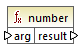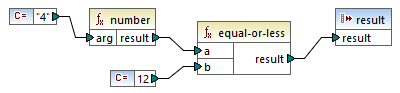# Altova MapForce 2022 Enterprise Edition

## number

Converts the value of arg into a number, where arg is a string or Boolean value. If arg is a string, MapForce will attempt to parse it as a number. For example, a string like "12.56" is converted to the decimal value 12.56. If arg is Boolean true, it is converted to numeric 1. If arg is Boolean false, it is converted to numeric 0.## Languages

Built-in, C++, C#, Java, XQuery, XSLT 1.0, XSLT 2.0, XSLT 3.0.

## Parameters

Argument

Description

arg

Mandatory argument. Supplies the value to be converted.

## Example

In the example below, the first constant is of type string and it contains the string "4". The second constant contains the numeric constant 12. In order for the two values to be compared as numbers, the types must agree.Adding a number function to the first constant converts the string "4" to the numeric value of 4. The result of the comparison is then "true". If the number function were not used (that is, if "4" was connected directly to a), a string comparison would occur, with the result being "false".